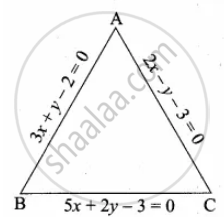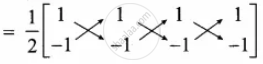Tamil Nadu Board of Secondary EducationSSLC (English Medium) Class 10th
Advertisement Remove all ads

# Find the area of a triangle formed by the lines 3x + y – 2 = 0, 5x + 2y – 3 = 0 and 2x – y – 3 = 0 - Mathematics

Sum

Find the area of a triangle formed by the lines 3x + y – 2 = 0, 5x + 2y – 3 = 0 and 2x – y – 3 = 0

Advertisement Remove all ads

#### Solution

3x + y = 2   ...(1)

5x + 2y = 3  ...(2)

2x – y = 3   ...(3)

Solve (1) and (2) to get the vertices B(1) × 2 ⇒                    6x + 2y = 4    ...(1)
(1) × 2 ⇒                    5x + 2y = 3   ...(2)
(−)  (−)    (−)
Subtract (1) and (2) ⇒ x         = 1

Substitute the value of x = 1 in (1)

3(1) + y = 2

y = 2 – 3 = – 1

The point B is (1, – 1)

Solve (2) and (3) to get the vertices C

(2) × 1 ⇒                    5x + 2y = 3    ...(2)
(3) × 2 ⇒                    4x – 2y = 6     ...(4)
Adding (2) and (4) ⇒ 9x         = 9
x         = 1

Substitute the value of x = 1 in (3)

2(1) – y = 3

⇒ – y = 3 – 2

– y = 1

⇒ y = – 1

The point C is (1, – 1)

Solve (1) and (3) to get the vertices A

3x + y = 2    ...(1)
2x – 2y = 3     ...(2)
By adding (1) and (2) ⇒ 5x         = 5

Substitute the value of x = 1 in (1)

3(1) + y = 2

y = 2 – 3 = – 1

The point A is (1, – 1)

The points A (1, – 1), B (1, – 1), C(1, – 1)

Area of ∆ABC = 1/2[x_1y_2 + x_2y_3 + x_3y_1 - (x_2y_1 + x_3y_2 + x_1y_3)]= 1/2 [-1 + (- 1)+ (- 1) - (- 1 + (- 1) + (- 1))]

= 1/2[-3 - (- 3)]

= 1/2[- 3 + 3]

= 1/2 xx 0 = 0

Area of the triangle = 0 sq. units.

Is there an error in this question or solution?
Advertisement Remove all ads

#### APPEARS IN

Samacheer Kalvi Mathematics Class 10 SSLC Tamil Nadu State Board
Chapter 5 Coordinate Geometry
Unit Exercise – 5 | Q 3 | Page 237
Advertisement Remove all ads
Advertisement Remove all ads
Share
Notifications

View all notifications

Forgot password?